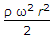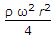# Mechanical Engineering - Hydraulics and Fluid Mechanics

### Exercise :: Hydraulics and Fluid Mechanics - Section 5

16.

The mass of 2.5 m3 of a certain liquid is 2 tonnes. Its mass density is

 A. 200 kg/m3 B. 400 kg/m3 C. 600 kg/m3 D. 800 kg/m3

Answer: Option D

Explanation:

No answer description available for this question. Let us discuss.

17.

The dynamic viscosity of the liquid __________ with rise in temperature.

 A. remain unaffected B. increases C. decreases

Answer: Option C

Explanation:

No answer description available for this question. Let us discuss.

18.

When a vertical wall is subjected to pressures due to liquid on both sides, the resultant pressure is the __________ of the two pressures.

 A. sum B. difference C. arithmatic mean D. geometric mean

Answer: Option B

Explanation:

No answer description available for this question. Let us discuss.

19.

The increase in pressure at the outer edge of a drum of radius (r) completely filled up with liquid of density (ρ) and rotating at (ω) rad/s is

 A. ρ ω2 r2 B. 2ρ ω2 r2 C.D.Answer: Option C

Explanation:

No answer description available for this question. Let us discuss.

20.

When the Mach number is between __________ the flow is called super-sonic flow.

 A. 1 and 2.5 B. 2.5 and 4 C. 4 and 6 D. 1 and 6

Answer: Option D

Explanation:

No answer description available for this question. Let us discuss.

#### Current Affairs 2021

Interview Questions and Answers